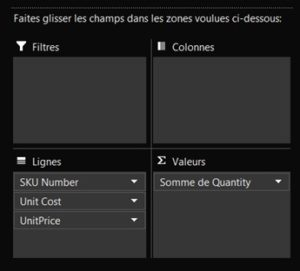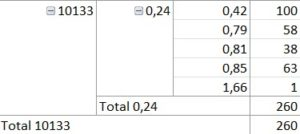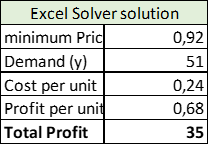# The easiest method to get a price optimization for your product in Excel

After using our beloved Excel for sales forecasting, it is now time to think about which pricing strategy to apply to maximize benefits. There’s plenty of methods but the one we’ll be discovering now is by far the easiest one as it does not require any Excel knowledge around formulas or math. Impressive?

Let’s give it a try.

We know you’re running out of time, so we’ve prepared an excel spreadsheet for you to follow this method with no big deal.

The dataset we used is a regular e-commerce sales historical data sample, where you’ll need to have those mandatory columns displayed – others you may have but also others in our example being optional:

• SKU Number
• Quantity sold
• Unit Price
• Unit cost

## II- Simple Excel prep before moving on

Now that you have the right columns, create a pivot table following the below parameters:• Nothing in the filters or columns tabs
• In the Rows tab, add in order : SKU Number / Unit Cost / Unit Price
• In Values, add the Quantity, change the parameters and set “SUM” to get the total number of items sold at a specific price point

You should then get the following for every item of your catalog:• 10133 being the SKU number
• 0,24 being the unit cost
• 0,42 to 1,66 being the different price points during the period of observation
• 100 to 1 being the quantities being sold depending on the applied price point

We’ll keep the item 10133 as the main reference for the rest of the analysis..

## III- Calculating the optimum price manually

In order to show you how powerful Excel can be, we’ll be getting the optimum price on our end first, using linear regression (y=ax+b).

To do so, select both quantities and price points and create a simple graph, adding the linear regression trend curve as well: click on Insert/Graph/curve, then click once on the graph and click on “add a graph element” on the top left corner, then on “trend curve” and “linear”. Repeat this sequence by clicking on “other opitons” right below “linear” in the last step to select “display the formula” in the options tab. This will reveal the exact parameters of the equation y=ax+b, showing how your sales tend to evolve when using linear regression.Knowing this equation, you can now simulate the optimum price using this formula. Considering prices and unit cost of item 10133, we get those results:• Minimum price is our (x)
• The demand is calculated (y) = -74,351*0,42+119,36
• Cost per unit is given (0,24)
• Profit per unit is price per unit – cost per unit
• Total profit is estimated demand (y) * profit per unit, meaning 88*0,18 = 16 (15,64 to be accurate)

## IV- Now what?

Be patient. Just for once. Cos we’ll know be using what Excel gave us all to go beyond our math weaknesses, you poor analytical thinkers (and doers as we all are). This simple, but smart tool is called “Solver”. It is displayed on the top right corner of the MS Pane under Data/Analysis. Don’t see it? No worries, nothing to install or to pay for, this is an embedded Excel add-in you must activate on the Excel options.

Ready? Then we shall start. Copy and paste the “linear regression solution” calculations you did in the same sheet. Only copy the values, not the formulas.

Then Click on Solver on the top right corner, in the Data/Analysis tab.1. Set Objective is the value we’d like to reach the maximum value of, in our case, we’d like to maximize our profit. In our example, you must select the “Total Profit” cell, currently reaching 16. Then Click on Max, obviously!
2. “By Changing variable cells”, meaning we’d like to maximize our profit by getting the best possible price so in our example we must select the “minimum price”, 0,42
3. “Subject to the constraints” gives you the ability to set the price boundaries. In our example, minimum price is 0,42 (and shouldn’t be lower) and maximum price is 1,66. You then have to add the following constraints :

a) 0,42 <= 1,66

b) 0,42 >= 0,42

4. Finally, choose the GRG non linear resolution (selected by default), as it is the most common one, do not pay attention to the others. And click on “solve”.

Once clicking on “solve”, Excel will kindly ask you if you want to change the values of your table by ticking the “keep solver solution”. Since this is a copy, you of course agree to change them – and you’ll be able to make a simple comparison afterwards with the linear regression values. Then click OK.

In our example, this is what we finally get:By suggesting a price around 0,92 for item 10133, the total profit is more than doubled with an expected total quantity to be sold reaching 51 units.

## V- What’s next?

Now you’ve got properly trained, you’ll be using this magic trick every time you need to update the pricing for a product. Read that sentence again. “For a product”. Yep. For a single one. In our data sample, we have more than 2800 products… meaning we’d have to go through all of these steps 2800 times. That’s the first thing.

Then, there’s one thing Excel does NOT consider that might have a significant impact on those results: TIME. What if I had to increase the price due to a material shortage, thus increasing the production costs? What if I can increase the price cos I’m the only one selling those in this country? It of course will have an impact on the demand on the short term, but definitely a strong positive profit impact on a mid-term perspective. That’s the second limitation we meet by using Excel for price optimization.

What if you’re dealing with those limitations (which will be the case eventually)? We’ll get you covered. Our marketplace offers an instant pricing optimization analysis, ready to be used with your own data in a few clicks – push your dataset, get yourself a coffee, and get your results delivered to your favorite software or a simple excel spreadsheet in no time!

No time to do it manually? Take a look at our free ready-to-use pricing optimization analysis: